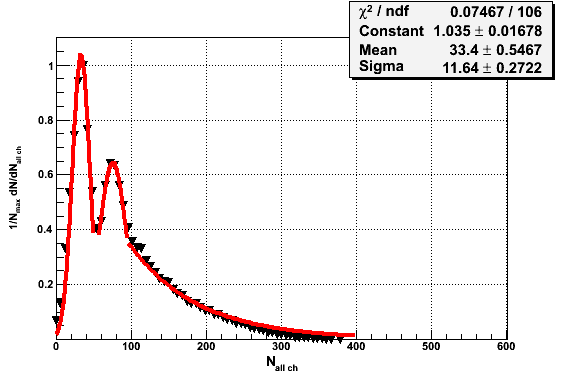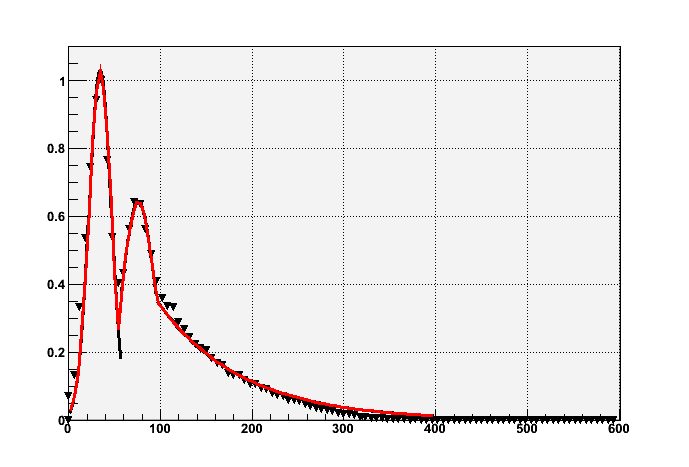# Smooth fit the graph with three separated fit function

Hello, i try to sew some deffent fit function, but i can’t do it. in ROOT example we see the file multifit.C (but it’s foк histo) . in this file total fit function is three gauss and it fit smoothly, but three separated gaus fit function doesn’t do it. so i ask for help in this question. how can i sew three separated fit function. thanks for response!

see \$ROOTSYS/tutorials/fit/FittingDemo.C

Rene

in that example fit by all fit function done on [0;3] . i ask about smooth fit which done by different function on different distance

Note that you can specify the fit range in the TH1::Fit function.

Rene

yes. i know. the result of fit is . it’s not smooth.Please clarify. You have a contradiction in “the result of fit is . it’s not smooth”

Rene

you see the picture. i try to do fit by three function: two gauss and exp(-x). and in boundary points i want to sew the curves: i do
TF1 * fun1=new TF1(“fun1”,“gaus”,0,57);
TF1 * fun2=new TF1(“fun2”,“gaus”,57,95);
TF1 * fun3=new TF1(“fun3”,myFun,95,400,1); and want that in the end of each range start curve flow to next curve. i hope , you understand me. thank you.

Two suggestions:
1- Increase the number of points where teh functions are computed

``` fun1->SetNpx(1000); fun2->SetNpx(1000); fun3->SetNpx(1000); ```
2- Define a global function like in multifit.C

[code] TF1 *fun1, *fun2, *fun3, *funtot;
double ftot(double *x, double *par) {
if (x < 57) {
return f1->EvalPar(x,par);
} else if (x < 95) {
return f2->EvalPar(x,&par);
} else {
return f3->EvalPar(x,\$par);
}
}

fun1=new TF1(“fun1”,“gaus”,0,57);
fun2=new TF1(“fun2”,“gaus”,57,95);
fun3=new TF1(“fun3”,myFun,95,400,1);
// fit individual functions then proceed like in multifit.C

double par;
fun1->GetParameters(&par);
fun2->GetParameters(&par);
fun3->GetParameters(&par);
TF1 *total = new TF1(“total”,ftot,0,400,3+3+n3); //where n3=number of parameters in ftot
total->SetParameters(par);
h->Fit(total,“R+”);
[/code]

Rene

thank you for you opinion, but it still doesn’t work.
what is the determination of EvalPar(x,par); function? maybe i’m doing something wrong?
fit_mn_Em.c (3.16 KB)

see modified file in attachment

Renefit_mn_Em.c (3.19 KB)